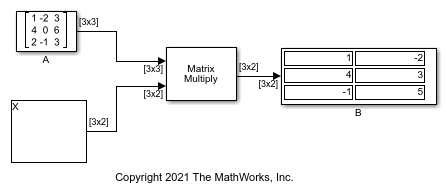# Solve Matrix Equation Using Singular Value Decomposition

Solve a matrix equation using the SVD Solver block.

Open and run the model. The model solves the equation AX = B using the SVD Solver block. The block uses A and B matrices as inputs and outputs the solution matrix X.You can verify the solution by using the Matrix Multiply block to perform the multiplication AX, as shown in the following model. The output matrix B in this model equals the input matrix B in the previous model.